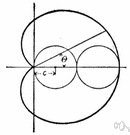# cardioid

(redirected from cardioid microphones)
Also found in: Thesaurus, Medical, Encyclopedia.
Related to cardioid microphones: unidirectional microphone
cardioid
The parametric equations of this cardioid are
x = -a cos θ (1 - cos θ),
y = a sin θ (1 - cos θ).

## car·di·oid

(kär′dē-oid′)
n.
A heart-shaped plane curve, the locus of a fixed point on a circle that rolls on the circumference of another circle with the same radius.

## cardioid

(ˈkɑːdɪˌɔɪd)
n
(Mathematics) a heart-shaped curve generated by a fixed point on a circle as it rolls around another fixed circle of equal radius, a. Equation: r = a(1 – cos φ), where r is the radius vector and φ is the polar angle
Collins English Dictionary – Complete and Unabridged, 12th Edition 2014 © HarperCollins Publishers 1991, 1994, 1998, 2000, 2003, 2006, 2007, 2009, 2011, 2014

## car•di•oid

(ˈkɑr diˌɔɪd)

n.
a heart-shaped curve traced by a point on a circle that rolls without slipping on another equal circle. Equation: r = a (1−cosA).
[1745–55; < Greek kardioeidḗs heart-shaped]
 Noun 1cardioid - an epicycloid in which the rolling circle equals the fixed circleepicycloid - a line generated by a point on a circle rolling around another circle